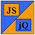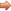# JavaScript & jQuery Tutorials

JS HomeJS AdvancedNumber

## Number

The `Number` global object allows us to wrap an object for mathematical use and comes with several useful properties and instance methods. In this lesson we look into `Number` creation and use some of the properties and methods available with this global object.

We can create our own `Number` objects using the `new` special operator or use `Number` in a non-constructor context to perform type conversion.

### Creating a `Number` object.

Let's look at an example of creating our own `Number` object and see some `Number` properties.

``````
// Create our own Number object.
function ourNumber(aValue) {
this.aValue = aValue;
}

// Inherit from the Number prototype.
ourNumber.prototype = new Number();
ourNumber.prototype.constructor = ourNumber;
// Min/Max Number Properties.

``````

Press the button below to action the above code:

### Finite and Infinite Numbers.

Let's look at examples of finite and infinite `Number` properties and test the `isFinite` global function against these.

``````
/*
* The isFinite method returns true for all values except
* +/- infinity and NaN.
*/
if (isFinite(Number.NEGATIVE_INFINITY)) {
} else if (isFinite(Number.POSITIVE_INFINITY)) {
} else if (isFinite(NaN)) {
} else {
}

// Infinity Properties.

``````

Press the button below to action the above code:

### Some `Number` Methods.

Let's look at examples of some `Number` methods.

``````
// The toExponential, toFixed and toLocaleString methods.
aNumber = new Number(1.23456789);

``````

Press the button below to action the above code:

### `Number` Type Conversion

We can also use `Number` in a non-constructor context for type conversion.

``````
// Adding a string and number concatenates them.
var aNumber = 12;
var bNumber = '12';
alert(aNumber + bNumber); // 1212 (concatenates)

// Using Number for type conversion.

``````

Press the button below to action the above code:

## Lesson 3 Complete

In this lesson we looked at the `Number` global object and some of its properties and methods.

## What's Next?

In the next lesson we look at the `Math` static global object and how to use it.